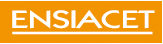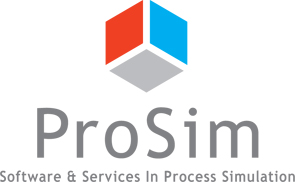On March 8, 2007, within the CAPE-OPEN 2007 Annual Meeting, Pascal FLOQUET from ENSIACET in Toulouse, with Xavier JOULIA also from ENSIACET and Alain VACHER, from ProSim SA, presented (PDF, 2291 Ko) experiments relying on CAPE-OPEN interoperability.P. FLOQUET expressed first the need for graphical representation of residue curve maps in order, for example, to better understand singular points, especially in a context of teaching thermodynamics and application of thermodynamics. The graphical capabilities of Matlab are well known and recognized. Hence P. FLOQUET felt the need to rely on such capabilities but Matlab is not including a thermodynamic server. So the idea came up of developing a link between Matlab and Simulis Thermodynamics so that the modelling within Matlab could access any CAPE-OPEN Property Package through the capabilities offered by Simulis Thermodynamics in terms of linkage through CAPE-OPEN.

So the application relies on three software tools: Matlab for the modelling and drawing of the residue curves, Simulis Thermodynamics for the layer between Matlab and a CAPE-OPEN Property Package, and a CAPE-OPEN Property Package, here one from TEA from AmsterCHEM, to provide thermodynamic calculations.

The application runs in three steps. First a CAPE-OPEN Property Package needs to be defined in TEA (it could be with another thermodynamic server capable of creating CAPE-OPEN Property Packages): this involves defining the compounds in the mixture as well as the properties to be calculated by the Property Package and the models to be used for calculating each property.

Next the application in Matlab that permits the drawing of residue curves needs to be developed as well as the part that calls for thermodynamic calculations through the layer provided by Simulis Thermodynamics. The last step involves running the application.

P. FLOQUET gave two examples of residue curve calculations, one with the methanol-ethanol-water mixture and one with acetone-methanol-chloroform mixture.

In terms of conclusive remarks, P. FLOQUET mentioned that CAPE-OPEN is about giving the ability to use the best tool for each project and here the application makes use of Matlab because it provides the best drawing capabilities while this or that thermodynamic server gives the best representation of this or that mixture. This leads to a vast reduction of development time, even for students since there is no need for them to know about CAPE-OPEN: they need to know about Matlab, about Simulis Thermodynamics and about COCO, that is about process modelling software, not programming.

Still the way the application worked was very time consuming, essentially because the number of similar calculations required are handled into loops rather than in one go.

P. FLOQUET is considering applying the same to reactive systems and also to see how a CAPE-OPEN Unit Operation may be used in Matlab.

Y07 4thCOEU ENSIACET